# Shape Construction Quiz I, Non Verbal Reasoning

## 25 Questions MCQ Test Non Verbal Reasoning | Shape Construction Quiz I, Non Verbal Reasoning

Description
Attempt Shape Construction Quiz I, Non Verbal Reasoning | 25 questions in 50 minutes | Mock test for LR preparation | Free important questions MCQ to study Non Verbal Reasoning for LR Exam | Download free PDF with solutions
QUESTION: 1

### Directions to Solve In each of the following questions, a set of five alternative figures 1, 2, 3, 4 and 5 followed by a set of four alternatives (A), (B), (C) and (D) is provided. It is required to select the alternative which represents three out of the five alternative figures which when fitted into each other would form a complete square. Question - Select the alternative which represents three out of the five alternative figures which when fitted into each other would form a complete square.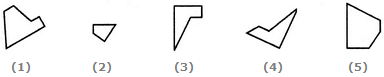Solution: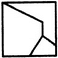QUESTION: 2

### Directions to Solve In each of the following questions, a set of five alternative figures 1, 2, 3, 4 and 5 followed by a set of four alternatives (A), (B), (C) and (D) is provided. It is required to select the alternative which represents three out of the five alternative figures which when fitted into each other would form a complete square. Question - Select the alternative which represents three out of the five alternative figures which when fitted into each other would form a complete square.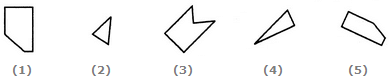Solution: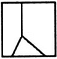QUESTION: 3

### Directions to Solve In each of the following questions, a set of five alternative figures 1, 2, 3, 4 and 5 followed by a set of four alternatives (A), (B), (C) and (D) is provided. It is required to select the alternative which represents three out of the five alternative figures which when fitted into each other would form a complete square. Question - Select the alternative which represents three out of the five alternative figures which when fitted into each other would form a complete square.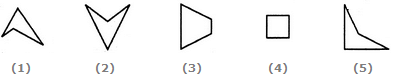Solution: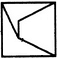QUESTION: 4

In each of the following questions, a set of five alternative figures 1, 2, 3, 4 and 5 followed by a set of four alternatives (A), (B), (C) and (D) is provided. It is required to select the alternative which represents three out of the five alternative figures which when fitted into each other would form a complete square.

Question -

Select the alternative which represents three out of the five alternative figures which when fitted into each other would form a complete square.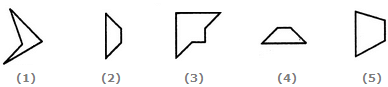Solution: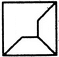QUESTION: 5

In each of the following questions, a set of five alternative figures 1, 2, 3, 4 and 5 followed by a set of four alternatives (A), (B), (C) and (D) is provided. It is required to select the alternative which represents three out of the five alternative figures which when fitted into each other would form a complete square.

Question -

Select the alternative which represents three out of the five alternative figures which when fitted into each other would form a complete square.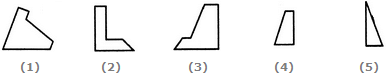Solution: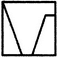QUESTION: 6

In each of the following questions, a set of five alternative figures 1, 2, 3, 4 and 5 followed by a set of four alternatives (A), (B), (C) and (D) is provided. It is required to select the alternative which represents three out of the five alternative figures which when fitted into each other would form a complete square.

Question -

Select the alternative which represents three out of the five alternative figures which when fitted into each other would form a complete square.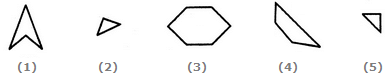Solution: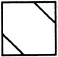QUESTION: 7

In each of the following questions, a set of five alternative figures 1, 2, 3, 4 and 5 followed by a set of four alternatives (A), (B), (C) and (D) is provided. It is required to select the alternative which represents three out of the five alternative figures which when fitted into each other would form a complete square.

Question -

Select the alternative which represents three out of the five alternative figures which when fitted into each other would form a complete square.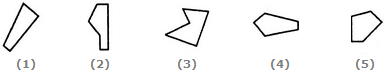Solution: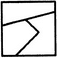QUESTION: 8

In each of the following questions, a set of five alternative figures 1, 2, 3, 4 and 5 followed by a set of four alternatives (A), (B), (C) and (D) is provided. It is required to select the alternative which represents three out of the five alternative figures which when fitted into each other would form a complete square.

Question -

Select the alternative which represents three out of the five alternative figures which when fitted into each other would form a complete square.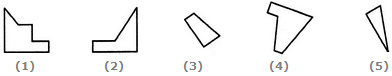Solution: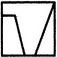QUESTION: 9

In each of the following questions, a set of five alternative figures 1, 2, 3, 4 and 5 followed by a set of four alternatives (A), (B), (C) and (D) is provided. It is required to select the alternative which represents three out of the five alternative figures which when fitted into each other would form a complete square.

Question -

Select the alternative which represents three out of the five alternative figures which when fitted into each other would form a complete square.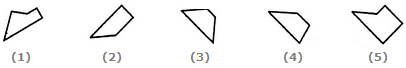Solution: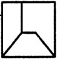QUESTION: 10

In each of the following questions, a set of five alternative figures 1, 2, 3, 4 and 5 followed by a set of four alternatives (A), (B), (C) and (D) is provided. It is required to select the alternative which represents three out of the five alternative figures which when fitted into each other would form a complete square.

Question -

Select the alternative which represents three out of the five alternative figures which when fitted into each other would form a complete square.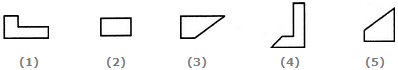Solution: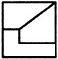QUESTION: 11

In each of the following questions, a set of five alternative figures 1, 2, 3, 4 and 5 followed by a set of four alternatives (A), (B), (C) and (D) is provided. It is required to select the alternative which represents three out of the five alternative figures which when fitted into each other would form a complete square.

Question -

Select the alternative which represents three out of the five alternative figures which when fitted into each other would form a complete square.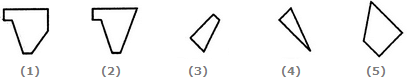Solution: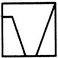QUESTION: 12

In each of the following questions, a set of five alternative figures 1, 2, 3, 4 and 5 followed by a set of four alternatives (A), (B), (C) and (D) is provided. It is required to select the alternative which represents three out of the five alternative figures which when fitted into each other would form a complete square.

Question -

Select the alternative which represents three out of the five alternative figures which when fitted into each other would form a complete square.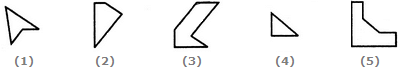Solution: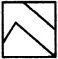QUESTION: 13

In each of the following questions, a set of five alternative figures 1, 2, 3, 4 and 5 followed by a set of four alternatives (A), (B), (C) and (D) is provided. It is required to select the alternative which represents three out of the five alternative figures which when fitted into each other would form a complete square.

Question -

Select the alternative which represents three out of the five alternative figures which when fitted into each other would form a complete square.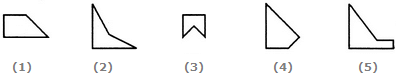Solution: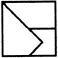QUESTION: 14

In each of the following questions, a set of five alternative figures 1, 2, 3, 4 and 5 followed by a set of four alternatives (A), (B), (C) and (D) is provided. It is required to select the alternative which represents three out of the five alternative figures which when fitted into each other would form a complete square.

Question -

Select the alternative which represents three out of the five alternative figures which when fitted into each other would form a complete square.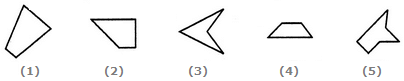Solution: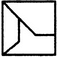QUESTION: 15

In each of the following questions, a set of five alternative figures 1, 2, 3, 4 and 5 followed by a set of four alternatives (A), (B), (C) and (D) is provided. It is required to select the alternative which represents three out of the five alternative figures which when fitted into each other would form a complete square.

Question -

Select the alternative which represents three out of the five alternative figures which when fitted into each other would form a complete square.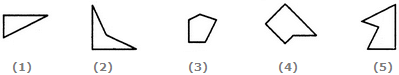Solution: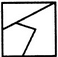QUESTION: 16

In each of the following questions, a set of five alternative figures 1, 2, 3, 4 and 5 followed by a set of four alternatives (A), (B), (C) and (D) is provided. It is required to select the alternative which represents three out of the five alternative figures which when fitted into each other would form a complete square.

Question -

Select the alternative which represents three out of the five alternative figures which when fitted into each other would form a complete square.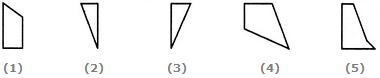Solution: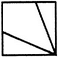QUESTION: 17

In each of the following questions, a set of five alternative figures 1, 2, 3, 4 and 5 followed by a set of four alternatives (A), (B), (C) and (D) is provided. It is required to select the alternative which represents three out of the five alternative figures which when fitted into each other would form a complete square.

Question -

Select the alternative which represents three out of the five alternative figures which when fitted into each other would form a complete square.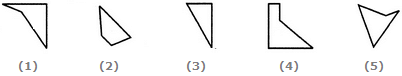Solution: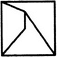QUESTION: 18

In each of the following questions, a set of five alternative figures 1, 2, 3, 4 and 5 followed by a set of four alternatives (A), (B), (C) and (D) is provided. It is required to select the alternative which represents three out of the five alternative figures which when fitted into each other would form a complete square.

Question -

Select the alternative which represents three out of the five alternative figures which when fitted into each other would form a complete square.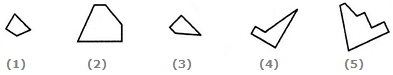Solution: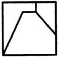QUESTION: 19

In each of the following questions, a set of five alternative figures 1, 2, 3, 4 and 5 followed by a set of four alternatives (A), (B), (C) and (D) is provided. It is required to select the alternative which represents three out of the five alternative figures which when fitted into each other would form a complete square.

Question -

Select the alternative which represents three out of the five alternative figures which when fitted into each other would form a complete square.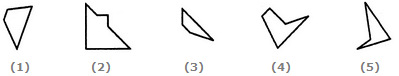Solution: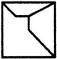QUESTION: 20

In each of the following questions, a set of five alternative figures 1, 2, 3, 4 and 5 followed by a set of four alternatives (A), (B), (C) and (D) is provided. It is required to select the alternative which represents three out of the five alternative figures which when fitted into each other would form a complete square.

Question -

Select the alternative which represents three out of the five alternative figures which when fitted into each other would form a complete square.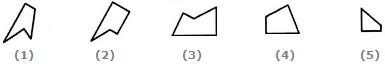Solution: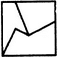QUESTION: 21

In each of the following questions, a set of five alternative figures 1, 2, 3, 4 and 5 followed by a set of four alternatives (A), (B), (C) and (D) is provided. It is required to select the alternative which represents three out of the five alternative figures which when fitted into each other would form a complete square.

Question -

Select the alternative which represents three out of the five alternative figures which when fitted into each other would form a complete square.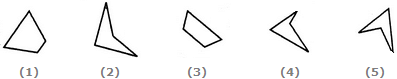Solution: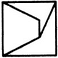QUESTION: 22

In each of the following questions, a set of five alternative figures 1, 2, 3, 4 and 5 followed by a set of four alternatives (A), (B), (C) and (D) is provided. It is required to select the alternative which represents three out of the five alternative figures which when fitted into each other would form a complete square.

Question -

Select the alternative which represents three out of the five alternative figures which when fitted into each other would form a complete square.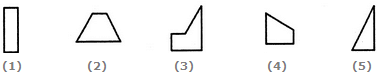Solution: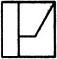QUESTION: 23

In each of the following questions, a set of five alternative figures 1, 2, 3, 4 and 5 followed by a set of four alternatives (A), (B), (C) and (D) is provided. It is required to select the alternative which represents three out of the five alternative figures which when fitted into each other would form a complete square.

Question -

Select the alternative which represents three out of the five alternative figures which when fitted into each other would form a complete square.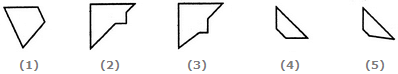Solution: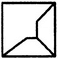QUESTION: 24

In each of the following questions, a set of five alternative figures 1, 2, 3, 4 and 5 followed by a set of four alternatives (A), (B), (C) and (D) is provided. It is required to select the alternative which represents three out of the five alternative figures which when fitted into each other would form a complete square.

Question -

Select the alternative which represents three out of the five alternative figures which when fitted into each other would form a complete square.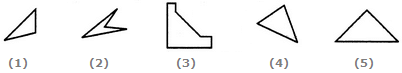Solution: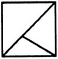QUESTION: 25

In each of the following questions, a set of five alternative figures 1, 2, 3, 4 and 5 followed by a set of four alternatives (A), (B), (C) and (D) is provided. It is required to select the alternative which represents three out of the five alternative figures which when fitted into each other would form a complete square.

Question -

Select the alternative which represents three out of the five alternative figures which when fitted into each other would form a complete square.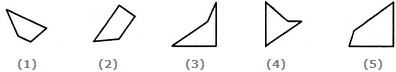Solution: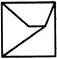Use Code STAYHOME200 and get INR 200 additional OFF Use Coupon Code